Discrete Mathematics and Combinatorics Commons™

945 Full-Text Articles 1,167 Authors 104,022 Downloads95 Institutions

All Articles in Discrete Mathematics and Combinatorics

945 full-text articles. Page 5 of 35.

Subsets Of Vertices Of The Same Size And The Same Maximum Distance, 2018Karlsruhe Institute of Technology

Subsets Of Vertices Of The Same Size And The Same Maximum Distance, Maria Axenovich, Dominik Duerrschnabel

Theory and Applications of Graphs

For a simple connected graph $G=(V,E)$ and a subset $X$ of its vertices, let $$d^*(X) = \max\{{\rm dist}_G(x,y): x,y\in X\}$$ and let

$h^*(G)$ be the largest $k$ such that there are disjoint vertex subsets $A$ and $B$ of $G$, each of size $k$ such that $d^*(A) = d^*(B).$

Let $h^*(n) = \min \{h^*(G): |V(G)|=n\}$. We prove that $h^*(n) = \lfloor (n+1)/3 \rfloor,$ for $n\geq 6.$ This solves the homometric set problem restricted to the largest distance exactly. In addition we compare $h^*(G)$ with ...

Pentagons In Triangle-Free Graphs, 2018Iowa State University

Pentagons In Triangle-Free Graphs, Bernard Lidicky, Florian Pfender

Mathematics Publications

For all n≥9, we show that the only triangle-free graphs on n vertices maximizing the number 5-cycles are balanced blow-ups of a 5-cycle. This completely resolves a conjecture by Erd\H{o}s, and extends results by Grzesik and Hatami, Hladky, Kral, Norin and Razborov, where they independently showed this same result for large n and for all n divisible by 5.

Italian Domination On Ladders And Related Products, 2018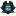East Tennessee State University

Italian Domination On Ladders And Related Products, Bradley Gardner

Electronic Theses and Dissertations

An Italian dominating function on a graph $G = (V,E)$ is a function such that $f : V \to \{0,1,2\}$, and for each vertex $v \in V$ for which $f(v) = 0$, we have $\sum_{u\in N(v)}f(u) \geq 2$. The weight of an Italian dominating function is $f(V) = \sum_{v\in V(G)}f(v)$. The minimum weight of all such functions on a graph $G$ is called the Italian domination number of $G$. In this thesis, we will consider Italian domination in various types of products of a graph $G$ with the complete ...

Finite Simple Graphs And Their Associated Graph Lattices, 2018Middle Tennessee State University

Finite Simple Graphs And Their Associated Graph Lattices, James B. Hart, Brian Frazier

Theory and Applications of Graphs

In his 2005 dissertation, Antoine Vella explored combinatorical aspects of finite graphs utilizing a topological space whose open sets are intimately tied to the structure of the graph. In this paper, we go a step further and examine some aspects of the open set lattices induced by these topological spaces. In particular, we will characterize all lattices that constitute the opens for finite simple graphs endowed with this topology, explore the structure of these lattices, and show that these lattices contain information necessary to reconstruct the graph and its complement in several ways.

Inducibility Of Directed Paths, 2018Hankuk University of Foreign Studies

Inducibility Of Directed Paths, Ilkyoo Choi, Bernard Lidicky, Florian Pfender

Mathematics Publications

A long standing open problem in extremal graph theory is to describe all graphs that maximize the number of induced copies of a path on four vertices. The character of the problem changes in the setting of oriented graphs, and becomes more tractable. Here we resolve this problem in the setting of oriented graphs without transitive triangles.

Erasure Coding For Distributed Matrix Multiplication For Matrices With Bounded Entries, 2018Iowa State University

Erasure Coding For Distributed Matrix Multiplication For Matrices With Bounded Entries, Li Tang, Konstantinos Konstantinidis, Aditya Ramamoorthy

Electrical and Computer Engineering Publications

Distributed matrix multiplication is widely used in several scientific domains. It is well recognized that computation times on distributed clusters are often dominated by the slowest workers (called stragglers). Recent work has demonstrated that straggler mitigation can be viewed as a problem of designing erasure codes. For matrices A and B, the technique essentially maps the computation of ATB into the multiplication of smaller (coded) submatrices. The stragglers are treated as erasures in this process. The computation can be completed as long as a certain number of workers (called the recovery threshold) complete their assigned tasks. We present a novel ...

Closing In On Hill's Conjecture, 2018University of Illinois at Urbana-Champaign

Closing In On Hill's Conjecture, JóZsef Balogh, Bernard Lidický, Gelasio Salazar

Bernard Lidický

Borrowing Laszlo Szekely's lively expression, we show that Hill's conjecture is asymptotically at least 98.5% true." This long-standing conjecture states that the crossing number cr(Kn) of the complete graph Kn is H(n) := 1 4 \lfloor n 2 \rfloor \lfloor n 1 2 \rfloor \lfloor n 2 2 \rfloor \lfloor n 3 2 \rfloor for all n \geq 3. This has been verified only for n \leq 12. Using the flag algebra framework, Norin and Zwols obtained the best known asymptotic lower bound for the crossing number of complete bipartite graphs, from which it follows that ...

2018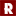Rose-Hulman Institute of Technology

A Proof Of The "Magicness" Of The Siam Construction Of A Magic Square, Joshua Arroyo

Rose-Hulman Undergraduate Mathematics Journal

A magic square is an n x n array filled with n2 distinct positive integers 1, 2, ..., n2 such that the sum of the n integers in each row, column, and each of the main diagonals are the same. A Latin square is an n x n array consisting of n distinct symbols such that each symbol appears exactly once in each row and column of the square. Many articles dealing with the construction of magic squares introduce the Siam method as a "simple'' construction for magic squares. Rarely, however, does the article actually prove that the construction ...

On Orders Of Elliptic Curves Over Finite Fields, 2018Columbia University

On Orders Of Elliptic Curves Over Finite Fields, Yujin H. Kim, Jackson Bahr, Eric Neyman, Gregory Taylor

Rose-Hulman Undergraduate Mathematics Journal

In this work, we completely characterize by $j$-invariant the number of orders of elliptic curves over all finite fields $F_{p^r}$ using combinatorial arguments and elementary number theory. Whenever possible, we state and prove exactly which orders can be taken on.

On The Largest Distance (Signless Laplacian) Eigenvalue Of Non-Transmission-Regular Graphs, 2018East China Normal University

On The Largest Distance (Signless Laplacian) Eigenvalue Of Non-Transmission-Regular Graphs, Shuting Liu, Jinlong Shu, Jie Xue

Let $G=(V(G),E(G))$ be a $k$-connected graph with $n$ vertices and $m$ edges. Let $D(G)$ be the distance matrix of $G$. Suppose $\lambda_1(D)\geq \cdots \geq \lambda_n(D)$ are the $D$-eigenvalues of $G$. The transmission of $v_i \in V(G)$, denoted by $Tr_G(v_i)$ is defined to be the sum of distances from $v_i$ to all other vertices of $G$, i.e., the row sum $D_{i}(G)$ of $D(G)$ indexed by vertex $v_i$ and suppose that $D_1(G)\geq \cdots \geq D_n(G)$. The $Wiener~ index$ of $G$ denoted by $W ... Spectral Bounds For The Connectivity Of Regular Graphs With Given Order, 2018Maastricht University Spectral Bounds For The Connectivity Of Regular Graphs With Given Order, Aida Abiad, Boris Brimkov, Xavier Martinez-Rivera, Suil O, Jingmei Zhang Electronic Journal of Linear Algebra The second-largest eigenvalue and second-smallest Laplacian eigenvalue of a graph are measures of its connectivity. These eigenvalues can be used to analyze the robustness, resilience, and synchronizability of networks, and are related to connectivity attributes such as the vertex- and edge-connectivity, isoperimetric number, and characteristic path length. In this paper, two upper bounds are presented for the second-largest eigenvalues of regular graphs and multigraphs of a given order which guarantee a desired vertex- or edge-connectivity. The given bounds are in terms of the order and degree of the graphs, and hold with equality for infinite families of graphs. These results ... Transformations On Double Occurrence Words Motivated By Dna Rearrangement, 2018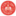University of South Florida Transformations On Double Occurrence Words Motivated By Dna Rearrangement, Daniel Cruz, Margherita Maria Ferrari, Lukas Nabergall, Natasa Jonoska, Masahico Saito Annual Symposium on Biomathematics and Ecology: Education and Research No abstract provided. 2018Illinois State University The Influence Of Canalization On The Robustness Of Finite Dynamical Systems, Claus Kadelka Annual Symposium on Biomathematics and Ecology: Education and Research No abstract provided. Combinatorial Geometry Of Threshold-Linear Networks, 2018Illinois State University Combinatorial Geometry Of Threshold-Linear Networks, Christopher Langdon Annual Symposium on Biomathematics and Ecology: Education and Research No abstract provided. Reducing The Maximum Degree Of A Graph By Deleting Vertices: The Extremal Cases, 2018University of Malta Reducing The Maximum Degree Of A Graph By Deleting Vertices: The Extremal Cases, Peter Borg, Kurt Fenech Theory and Applications of Graphs Let$\lambda(G)$denote the smallest number of vertices that can be removed from a non-empty graph$G$so that the resulting graph has a smaller maximum degree. In a recent paper, we proved that if$n$is the number of vertices of$G$,$k$is the maximum degree of$G$, and$t$is the number of vertices of degree$k$, then$\lambda (G) \leq \frac{n+(k-1)t}{2k}$. We also showed that$\lambda (G) \leq \frac{n}{k+1}$if$G\$ is a tree. In this paper, we provide a new proof of the first bound and use ...

Using Magic In Computing Education And Outreach, 2018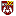Loyola University Chicago

Using Magic In Computing Education And Outreach, Ronald I. Greenberg, Dale F. Reed

Computer Science: Faculty Publications and Other Works

This special session explores the use of magic tricks based on computer science ideas; magic tricks help grab students' attention and can motivate them to invest more deeply in underlying CS concepts. Error detection ideas long used by computer scientists provide a particularly rich basis for working such "magic'', with a CS Unplugged parity check activity being a notable example. Prior work has shown that one can perform much more sophisticated tricks than the relatively well-known CS Unplugged activity, and these tricks can motivate analyses across a wide variety of computer science concepts and are relevant to learning objectives across ...

Bounds On The Sum Of Minimum Semidefinite Rank Of A Graph And Its Complement, 2018Central Michigan University

Bounds On The Sum Of Minimum Semidefinite Rank Of A Graph And Its Complement, Sivaram Narayan, Yousra Sharawi

Electronic Journal of Linear Algebra

The minimum semi-definite rank (msr) of a graph is the minimum rank among all positive semi-definite matrices associated to the graph. The graph complement conjecture gives an upper bound for the sum of the msr of a graph and the msr of its complement. It is shown that when the msr of a graph is equal to its independence number, the graph complement conjecture holds with a better upper bound. Several sufficient conditions are provided for the msr of different classes of graphs to equal to its independence number.

Microstructure Design Using Graphs, 2018Iowa State University

Microstructure Design Using Graphs, Pengfei Du, Adrian Zebrowski, Jaroslaw Zola, Baskar Ganapathysubramanian, Olga Wodo

Mechanical Engineering Publications

Thin films with tailored microstructures are an emerging class of materials with applications such as battery electrodes, organic electronics, and biosensors. Such thin film devices typically exhibit a multi-phase microstructure that is confined, and show large anisotropy. Current approaches to microstructure design focus on optimizing bulk properties, by tuning features that are statistically averaged over a representative volume. Here, we report a tool for morphogenesis posed as a graph-based optimization problem that evolves microstructures recognizing confinement and anisotropy constraints. We illustrate the approach by designing optimized morphologies for photovoltaic applications, and evolve an initial morphology into an optimized morphology exhibiting ...

2018Rose-Hulman Institute of Technology

Stranded Cellular Automaton And Weaving Products, Hao Yang

Mathematical Sciences Technical Reports (MSTR)

In order to analyze weaving products mathematically and find out valid weaving products, it is natural to relate them to Cellular Automaton. They are both generated based on specific rules and some initial conditions. Holden and Holden have created a Stranded Cellular Automaton that can represent common weaving and braiding products. Based on their previous findings, we were able to construct a Java program and analyze various aspects of the automaton they created. This paper will discuss the complexity of the Stranded Cellular Automaton, how to determine whether a weaving product holds together or not based on the automaton and ...

Tutte-Equivalent Matroids, 2018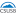California State University - San Bernardino

Tutte-Equivalent Matroids, Maria Margarita Rocha

Electronic Theses, Projects, and Dissertations

We begin by introducing matroids in the context of finite collections of vectors from a vector space over a specified field, where the notion of independence is linear independence. Then we will introduce the concept of a matroid invariant. Specifically, we will look at the Tutte polynomial, which is a well-defined two-variable invariant that can be used to determine differences and similarities between a collection of given matroids. The Tutte polynomial can tell us certain properties of a given matroid (such as the number of bases, independent sets, etc.) without the need to manually solve for them. Although the Tutte ...